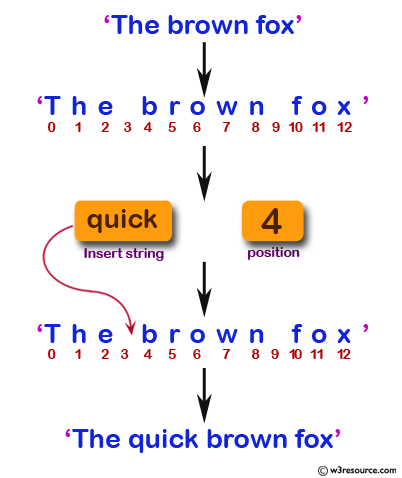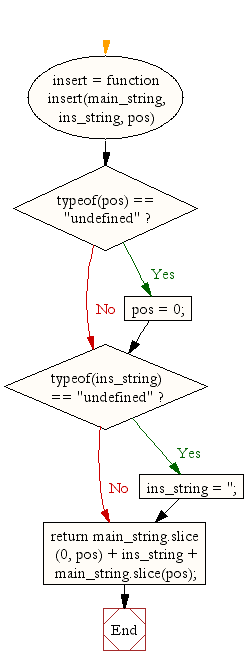# JavaScript: Insert a string within a specific position in another string

## JavaScript String: Exercise-14 with Solution

Write a JavaScript function to insert a string within a string at a particular position (default is 1).

Test Data:
console.log(insert('We are doing some exercises.'));
console.log(insert('We are doing some exercises.','JavaScript '));
console.log(insert('We are doing some exercises.','JavaScript ',18));
"We are doing some exercises."
"JavaScript We are doing some exercises."
"We are doing some JavaScript exercises."

Pictorial Presentation:Sample Solution:-

HTML Code:

``````<!DOCTYPE html>
<html>
<meta charset="utf-8">
<title>Insert a string within a specific position in another string</title>
<body>
</body>
</html>
```
```

JavaScript Code:

``````insert = function insert(main_string, ins_string, pos) {
if(typeof(pos) == "undefined") {
pos = 0;
}
if(typeof(ins_string) == "undefined") {
ins_string = '';
}
return main_string.slice(0, pos) + ins_string + main_string.slice(pos);
}
console.log(insert('We are doing some exercises.'));
console.log(insert('We are doing some exercises.','JavaScript '));
console.log(insert('We are doing some exercises.','JavaScript ',18));
```
```

Sample Output:

```We are doing some exercises.
JavaScript We are doing some exercises.
We are doing some JavaScript exercises.
```

Flowchart:Live Demo:

See the Pen JavaScript Insert a string within a specific position in another string - string-ex-14 by w3resource (@w3resource) on CodePen.

Improve this sample solution and post your code through Disqus

What is the difficulty level of this exercise?

Test your Programming skills with w3resource's quiz.

﻿

## JavaScript: Tips of the Day

Returns true if the given number is a power of 2, false otherwise

Example:

```const tips_isPowerOfTwo = n => !!n && (n & (n - 1)) == 0;
console.log(tips_isPowerOfTwo(0));
console.log(tips_isPowerOfTwo(4));
console.log(tips_isPowerOfTwo(16));
```

Output:

```false
true
true
```